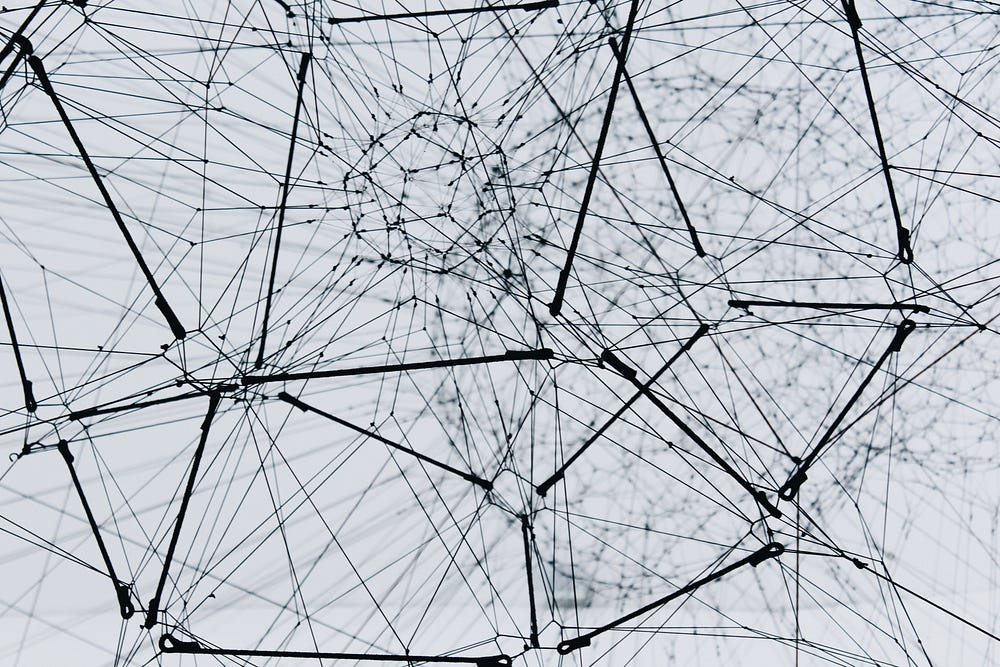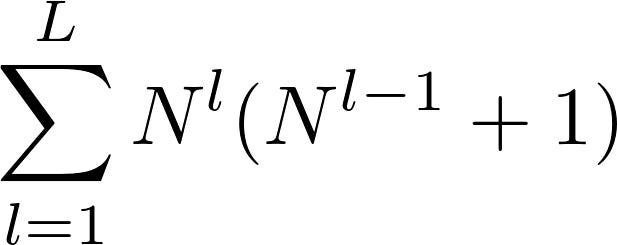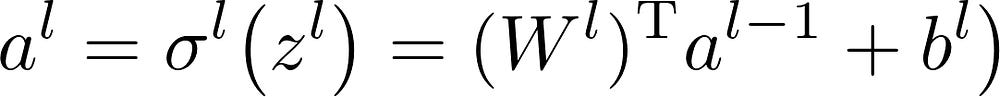# Machine Learning in Python NumPy: Neural Network in 9 Steps

Source: Deep Learning on Medium

## Understanding neural networks by codingPhoto by Alina Grubnyak on Unsplash

### Motivation

If you are a junior data scientist who sort of understands how neural nets work, or a machine learning enthusiast who only knows a little about deep learning, this is the article that you cannot miss. Here is how you can build a neural net from scratch using NumPy in 9 steps — from data pre-processing to back-propagation — a must-do practice.

Basic understanding of machine learning, artificial neural network, Python syntax, and programming logic is preferred (but not necessary as you can learn on the go).

Codes are available on Github. Originally published at edenau.github.io.

### 1. Initialization

Step one. Import NumPy. Seriously.

### 2. Data Generation

Deep learning is data-hungry. Although there are many clean datasets available online, we will generate our own for simplicity — for inputs a and b, we have outputs a+b, a-b, and |a-b|. 10,000 datum points are generated.Photo by Kristopher Roller on Unsplash

### 3. Train-test Splitting

Our dataset is split into training (70%) and testing (30%) set. Only training set is leveraged for tuning neural networks. Testing set is used only for performance evaluation when the training is complete.

### 4. Data Standardization

Data in the training set is standardized so that the distribution for each standardized feature is zero-mean and unit-variance. The scalers generated from the abovementioned procedure can then be applied to the testing set.These lines might look intimidating but they are quite repetitive.

The scaler therefore does not contain any information from our testing set. We do not want our neural net to gain any information regarding testing set before network tuning.

We have now completed the data pre-processing procedures in 4 steps.

### 5. Neural Net Construction

We objectify a ‘layer’ using class in Python. Every layer (except the input layer) has a weight matrix W, a bias vector b, and an activation function. Each layer is appended to a list called neural_net. That list would then be a representation of your fully connected neural network.

Finally, we do a sanity check on the number of hyperparameters using the following formula, and by counting. The number of datums available should exceed the number of hyperparameters, otherwise it will definitely overfit.N^l is number of hyperparameters at l-th layer, L is number of layers (excluding input layer)

### 6. Forward Propagation

We define a function for forward propagation given a certain set of weights and biases. The connection between layers is defined in matrix form as:σ is element-wise activation function, superscript T means transpose of a matrix

Activation functions are defined one by one. ReLU is implemented as a → max(a,0), whereas sigmoid function should return a → 1/(1+e^(-a)), and its implementation is left as an exercise to the reader.

### 7. Back-propagation

This is the most tricky part where many of us simply do not understand. Once we have defined a loss metric e for evaluating performance, we would like to know how the loss metric change when we perturb each weight or bias.

We want to know how sensitive each weight and bias is with respect to the loss metric.

This is represented by partial derivatives ∂e/∂W (denoted dW in code) and ∂e/∂b (denoted db in code) respectively, and can be calculated analytically.

These back-propagation equations assume only one datum y is compared. The gradient update process would be very noisy as the performance of each iteration is subject to one datum point only. Multiple datums can be used to reduce the noise where ∂W(y_1, y_2, …) would be the mean of ∂W(y_1), ∂W(y_2), …, and likewise for ∂b. This is not shown above in those equations, but are implemented in the code below.

### 8. Iterative Optimization

We now have every building block for training a neural network.

Once we know the sensitivities of weights and biases, we try to minimize (hence the minus sign) the loss metric iteratively by gradient descent using the following update rule:

`∂W = ∂W - learning_rate * ∂W∂b = ∂b - learning_rate * ∂b`Photo by Rostyslav Savchyn on UnsplashTraining loss should be going down as it iterates

### 9. Testing

The model generalizes well if the testing loss is not much higher than the training loss. We also make some test cases to see how the model performs.

### The Takeaway

This is how you can build a neural net from scratch using NumPy in 9 steps. Some of you might have already built neural nets using some high-level frameworks such as TensorFlow, PyTorch, or Keras. However, building a neural net using only low-level libraries enable us to truly understand the mathematics behind the mystery.

My implementation by no means is the most efficient way to build and train a neural net. There is so much room for improvement but that is a story for another day. Codes are available on Github. Happy coding!

#### Related Articles

Thank you for reading! If you are interested in machine learning or Python, check out the following articles:

Originally published at edenau.github.io.